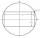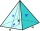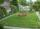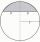# Area of shape + Pythagorean theorem - math problems

#### Number of problems found: 213

• Area of a rectangleCalculate the area of a rectangle with a diagonal of u = 12.5cm and a width of b = 3.5cm. Use the Pythagorean theorem.
• Annular areaThe square with side a = 1 is inscribed and circumscribed by circles. Find the annular area.
• Inscribed rectangleThe circle area is 216. Determine the area of inscribed rectangle with one side 5 long.
• The plaster castThe plaster cast has the shape of a regular quadrilateral pyramid. The cover consists of four equilateral triangles with a 5 m side. Calculate its volume and surface area.
• Garden fenceThe garden has the shape of a rectangular triangle with an area of 96 square meters and a 16 m long one leg. How many meters of the fence need to be fenced?
• Diagonal to areaCalculate the area of a rectangle in which the length of the diagonal is 10 cm.
• SquareCalculate the area of the square shape of the isosceles triangle with the arms 50m and the base 60m. How many tiles are used to pave the square if the area of one tile is 25 dm2?
• SquareCalculate area of the square with diagonal 64 cm.
• Equilateral triangle v2Equilateral triangle has a perimeter 36 dm. What is its area?
• CirclesArea of circle inscribed in a square is 14. What is the area of a circle circumscribed around a square?
• Spherical capWhat is the surface area of a spherical cap, the base diameter 20 m, height 2 m.
• RhombusThe rhombus with area 68 has one diagonal is longer by 6 than second one. Calculate the length of the diagonals and rhombus sides.
• Equilateral triangleCalculate the area of an equilateral triangle with circumference 72cm.
• Equilateral triangleThe equilateral triangle has a 23 cm long side. Calculate its content area.
• TriangleCalculate the sides of the triangle if its area S = 630 and the second cathethus is shorter by 17.
• SquareCalculate the perimeter and the area of square with a diagonal length 30 cm.
• Equilateral triangleCalculate the side of an equilateral triangle, if its area is 892 mm2.
• Hexagon areaThe center of the regular hexagon is 21 cm away from its side. Calculate the hexagon side and its area.
• AirplaneAviator sees part of the earth's surface with an area of 200,000 square kilometers. How high he flies?
• Is right triangle or notIf right triangle ABC, have sides a=13, b=11.5, c=22.5. Find area.

Do you have an interesting mathematical word problem that you can't solve it? Submit a math problem, and we can try to solve it.

We will send a solution to your e-mail address. Solved examples are also published here. Please enter the e-mail correctly and check whether you don't have a full mailbox.

Please do not submit problems from current active competitions such as Mathematical Olympiad, correspondence seminars etc...

Pythagorean theorem is the base for the right triangle calculator. Examples of area of plane shapes. Pythagorean theorem - math word problems.